Gibbs distribution

Ref 1 Eq. 3.37: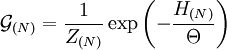$\mathcal{G}_{(N)} = \frac{1}{Z_{(N)}} \exp \left( - \frac{H_{(N)}}{\Theta}\right)$

where$N$ is the number of particles,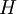$H$ is the Hamiltonian of the system and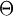$\Theta$ is the temperature (to convert$\Theta$ into the more familiar kelvin scale one divides by the Boltzmann constant$k_B$). The constant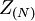$Z_{(N)}$ is found from the normalization condition (Ref. 1 Eq. 3.38)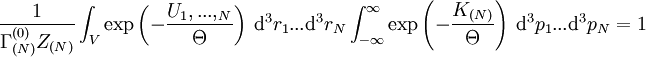$\frac{1}{\Gamma_{(N)}^{(0)}Z_{(N)}} \int_V \exp \left( - \frac{U_1,..., _N}{\Theta}\right) ~{\rm d}^3r_1 ... {\rm d}^3r_N \int_{- \infty}^{\infty} \exp \left( - \frac{K_{(N)}}{\Theta}\right) ~{\rm d}^3p_1 ... {\rm d}^3p_N =1$

which leads to (Ref. 1 Eq. 3.40)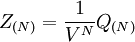$Z_{(N)}= \frac{1}{V^N} Q_{(N)}$

where (Ref. 1 Eq. 3.41)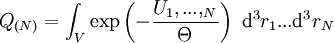$Q_{(N)} = \int_V \exp \left( - \frac{U_1,..., _N}{\Theta}\right) ~{\rm d}^3r_1 ... {\rm d}^3r_N$

this is the statistical integral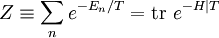$Z \equiv \sum_n e^{-E_n/T}= {\rm tr} ~ e^{-H|T}$

where$H$ is the Hamiltonian of the system.